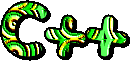# User-Defined Functionsc++ snippets

Q1: Write a function to return the largest number among three integers

```// function to return the largest number
// among three integers
// function declaration
int largest_num(int , int, int);

#include <iostream.h>
#include <conio.h>

int main()
{
// clear the screen
clrscr();
int x, y, z;
cout << "enter 1st no.=";
cin >> x;
cout << "enter 2nd no.=";
cin >> y;
cout << "enter 3rd no.=";
cin >> z;
cout << "largest of three is=" <<
largest_num(x, y, z);
getch();
return 0;
}

// function largest_num
// input three integers
// output: returns largest number.
int largest_num(int a, int b, int c)
{
int max;
if (a>b)
{
max = a;
}
else
max = b;
if (max < c )
{
max = c;
}
return max;
}
```

Q2: What is a function definition?

Answer: A function definition describes how the function computes the value it returns. It is a declaration about the function. It is also called a function prototype. In general, it is defined before using the function, within the source file or in header (.h/.hpp) files.

Q3(Text Book): Write a C++ program that reads a float array having 15 elements. The program uses a function reverse( ) to reverse this array. Make suitable assumptions wherever required.

```// // program to reverse an array
//

#include <iostream.h>
#include <conio.h>

// functions declaration
void reverse(float arry[], int size);
void display(float arry[], int size);

int main()
{
// clear the screen
clrscr();
float flist = {12.2, 8.1, 6.75,
18.25, 30.18, 12.5, 56.0,
78.12, 10.24, 13.12, 34.65,
16.80, 12.23,
32.5, 11.25};
display(flist, 15);
cout <<"\nReversing the Array...\n";
reverse(flist, 15);
display(flist, 15);
getch();
return;
}

void display(float arry[], int size)
{
cout << "The list is:\n\t";
for (int i = 0; i < size; ++i)
cout << arry[i] << ", ";
cout << "\n";
}

void reverse(float arry[], int size)
{
float temp;
for (int i = 0; i <= size/2; ++i)
{
temp = arry[i];
arry[i] = arry[size - i -1];
arry[size - i - 1] = temp;
}
}
```

Q4(Text Book): Write a complete C++ program that invokes a function satis( ) to find whether four integer a,b,c,d sent to satis( ) satisfy the equation a3 + b3 + c3 = d3 or not. The function satis( ) returns 0 if the above equation is satisfied with the given four numbers otherwise it returns -1.

```// function satis()
/* wap to find whether 4 integers
a,b,c,d sent to satis()
satisfy the
eq. a^3 + b^3 + c^3 = d^3*/
//

#include <iostream.h>
#include <math.h>
#include <conio.h>

// functions declaration
int satis(int, int, int, int);

void main()
{
clrscr();
cout << "condition for F(2,3,4,6)=";
if (satis(2,3,4,5) == 0 )
cout << "true";
else
cout << "false";
cout << "\n\nCondition for
F(6,8,10,12)=";
if (satis(6,8,10,12) == 0 )
cout << "true";
else
cout << "false";

cout << endl;
getch();
}

int satis(int a, int b, int c, int d)
{
if ((pow(a, 3) + pow(b,3) +
pow(c,3)) == pow(d,3))
return 0;
return -1;
}

```

Q5(Text Book): Write a C++ program that uses the following functions:
(i) sqlarge( ) that is passed two int arguments by reference and then sets the larger of the two numbers to its square.
(ii) sum( ) that is passed an int argument by value and that returns the sum of the individual digits of the passed number.
(iii) main( ) that exercises the above two functions by getting two integers from the user and by printing the sum of the individual digits of the square of the larger number.

```// functions
/*wap that uses the function sqlarge()
that is passed two int arguments by
reference & then sets the
larger of the two nos.to its square*/
//

#include <iostream.h>
#include <conio.h>

// functions declaration
void sqlarge(int &, int &);
int sum(int num);

void main()
{
int first, second, sqnum;
clrscr();
cout << "Enter first number: ";
cin >> first;
cout << "Enter second number: ";
cin >> second;
sqlarge(first, second);
if (first > second)
sqnum = first;
else
sqnum = second;
cout << "Square of large number = "
<< sqnum;
cout << "\nSum of digits of
square number = " << sum(sqnum);

getch();

}

void sqlarge(int &a, int &b)
{
if (a > b)
a = a * a;
else
b = b * b;
}

int sum(int num)
{
int s = 0;
while (num > 0)
{
s += (num % 10);
num = num / 10;
}
return s;
}

```
Q6: Write a c++ function swap( ) to swap to integers passed to the function.

```// // function swap() to
swap two numbers
//

#include <iostream.h>
#include <conio.h>

// functions declaration
void swap(int& x, int & y);

void main()
{
clrscr();
clrscr();
int a,b;
cout << "enter first no.=";
cin >> a;
cout <<"enter second no.=";
cin >> b;
cout << "1st No. (before swap)="
<< a;
cout << "\n2nd No. (before swap)="
<< b;
swap(a, b);
cout << "\n1st Number (after swap)="
<< a;
cout << "\n2nd number (after swap)="
<< b;
getch();
return 0;
}

// swap(), arguments passed as call
by references.
void swap(int& x, int & y)
{
x = x + y;
y = x - y;
x = x - y;
}

```

.